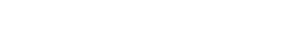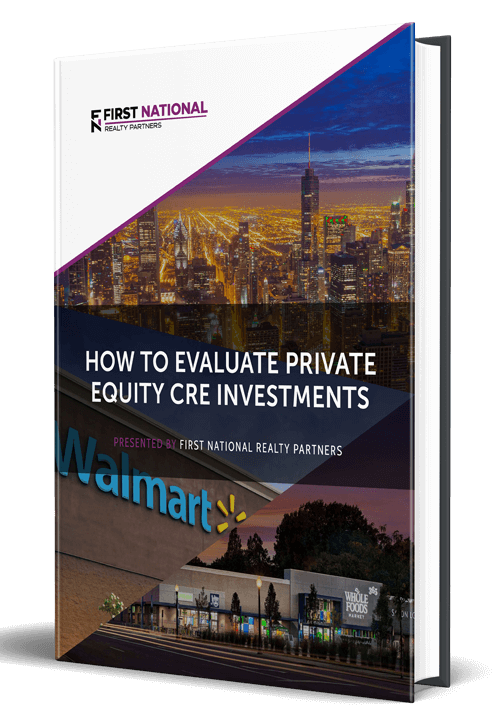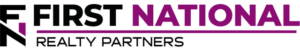# What Is the Terminal Cap Rate in Commercial Real Estate? Overview & Formula

### Key Takeaways

• The terminal cap rate is an important real estate metric that is defined as the cap rate applied to a property’s cash flow in the final year of the real estate investment holding period.
• The terminal cap rate is calculated as the net operating income in the year of sale divided by the property’s value.  If the value isn’t known, an estimate of the terminal cap rate based on sales of similar properties can be applied to final year net operating income to get it.
• The terminal cap rate is important for two reasons.  First, it can be used to calculate the terminal value/sales price.  Second, the resulting value can be used to calculate real estate investment return metrics, which are an important input into the ultimate decision of whether or not to purchase a property.

For most commercial real estate investments, the general strategy is the same.  The idea is to purchase a property, hold it for some period of time, and then sell it for more than the purchase price.  When creating a financial profitability model for this investment scenario, certain assumptions must be made to estimate the property’s sales price.  There are several methodologies that can be used to justify sales assumptions in commercial real estate, one of which is to apply a “terminal cap rate” to the property’s net operating income (NOI) in the year of sale.

In this article, we define the terminal cap rate, explain how to calculate the terminal cap rate, and provide an example for how it can be used to calculate the value of a property.  By the end of the article, readers will have a greater understanding of what the terminal cap rate real estate metric is and how it can be used in financial modeling.

At First National Realty Partners, we invest a significant amount of time and resources in creating financial models for each of our properties and they typically include a terminal cap rate assumption.  We view these models as a key input into the decision to acquire a property.  To learn more about our current real estate investment opportunities, click here.

## What Is the Terminal Cap Rate?

Terminal cap rate, sometimes called the exit cap rate, is an important real estate metric that is defined as the cap rate applied to a property’s cash flow in the final year of the investment holding period.

Terminal cap rate is a term that can be explained in two parts. The word “terminal” refers to the fact that this is a metric that is applied at the end of the holding period. Capitalization rate, or cap rate for short, is a versatile metric that is used to refer to the relationship between an investment property’s net operating income (NOI) and its market value.

As a result, there are two important pieces of information that can be learned from reviewing it:

1. It provides an indication of the potential return on investment assuming the property is purchased with cash.
2. It can also be used as a way to assess the market’s opinion of the risk associated with the property.  If the market believes that the property has higher risk, it will have a higher cap rate/lower purchase price.  Conversely if the market judges the property to have a lower level of risk, it will have a lower cap rate/higher purchase price.

For both of these reasons, the terminal cap rate/terminal value has a significant impact on the expected net profitability in a real estate investment.

## How to Calculate the Terminal Cap Rate

The formula used to calculate the terminal cap rate is final year NOI divided by value:

Terminal Cap Rate = Final Year Net Operating Income / Value

The key difference between the terminal cap rate calculation and the “regular” cap rate calculation is that the terminal cap rate requires net operating income in the final year of the investment holding period.  This is obtained by creating a pro forma projection of income and operating expenses  from which net operating income can be calculated.

In the denominator, estimating the value of the property five or ten years into the future can be challenging. If the value is unavailable, it can be calculated by dividing the net operating income by an estimate of the terminal cap rate, which is based on sales of comparable properties.

## Why Is the Terminal Cap Rate Important?

The terminal cap rate is important for two reasons.

### 1. Calculating Terminal Value

First, the terminal cap rate is an input that is required to calculate the terminal value of a property.  The terminal cap rate equation above (Final Year NOI/Value) can be rearranged to do this so that:

Terminal Value = Final Year Net Operating Income / Terminal Cap Rate

For example, suppose that a property is estimated to have final year net operating income of \$500,000 and a terminal cap rate of 7% (based on sales of comparable properties).  In this scenario, the property has a terminal value of \$7.1MM (\$500,000 / 7%).  Once the terminal value is known, the second reason for the terminal cap rate’s importance becomes clear.

### 2. Calculating Profitability Metrics

With the terminal value, real estate investors can calculate profitability metrics like the internal rate of return (IRR), equity multiple, and net present value of a future income stream with a given discount rate.  In short, the terminal cap rate – and the resulting terminal value – is needed to get a full picture of a real estate investment’s potential performance.

## What is a Good Terminal Cap Rate?

Like many things in real estate investing, the definition of a “good” cap rate is relative.  Unfortunately, there is no number that an investor could point to and say “this is a good terminal cap rate.”  Instead, the assessment of whether a terminal cap rate is good is highly dependent upon a variety of factors including:

• growth rate of future cash flows,
• anticipated market rents at the time of sale,
• vacancy rates,
• property type, and
• market conditions.

As such, the closest a real estate investor can get to a “good” terminal cap rate is one that is close to those obtained in historical sales of comparative properties, adjusted for expected market conditions at the time of sale.

For example, if the cap rates for historical commercial property sales are 6.5%, but the estimated terminal cap rate for a potential investment is 5%, this is a likely indication that the estimated sale price is too high.  But, if the market average is 6.5% and the estimated terminal cap rate is 6.6%, the real estate investor has a stronger case that their terminal value is supported by the current and historical market.

## Summary & Conclusions

The terminal cap rate is an important real estate metric that is defined as the cap rate applied to a property’s cash flow in the final year of the investment holding period.

The terminal cap rate is calculated as the net operating income in the year of sale divided by the property’s value.  If the value isn’t known, an estimate of the terminal cap rate based on sales of similar properties can be applied to final year net operating income to get it.

The terminal cap rate is important for two reasons.  First, it can be used to calculate the terminal value/sales price.  Second, the resulting value can be used to calculate other real estate investment return metrics, which are an important input into the ultimate decision of whether or not to purchase a property.

## Interested In Learning More?

First National Realty Partners is one of the country’s leading private equity commercial real estate investment firms. With an intentional focus on finding world-class, multi-tenanted assets well below intrinsic value, we seek to create superior long-term, risk-adjusted returns for our investors while creating strong economic assets for the communities we invest in.

Get instant access to all of our current and past commercial real estate deals.## Subscribe Now

Get the latest news on real estate

1031 Exchange

Acquisitions

CRE Basics

## Get More From FNRP## How to Evaluate Private Equity CRE Investments## How to Complete a 1031 Exchange with a Private Equity Sponsor